# HCF - Highest Common Factor

HCF - Highest Common Factor
Go back to  'Numbers'

Explore the world of HCF by going through its various aspects and properties. Find answers to questions like what is the highest common factor for a group of numbers, easy ways to calculate HCF, its relation with LCM, and discover more interesting facts around them.

The Highest Common Factor (HCF) of two numbers is the largest possible number which divides both the numbers exactly.

##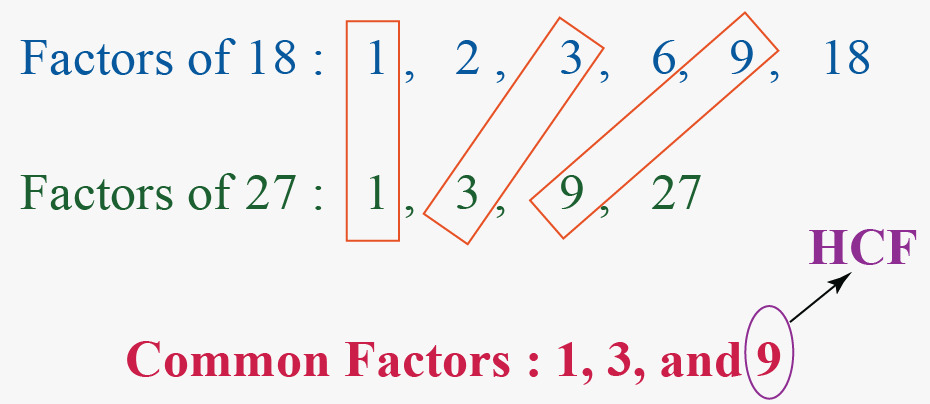Do you see how 9 is the Highest Common Factor of 18 and 27?

 1 What does HCF mean? 2 Learn These Along with HCF! 3 Solved Examples on HCF 4 Interactive Questions on HCF 5 FREE Worksheets on HCF

## What does HCF mean?

Before learning how to find the Highest Common Factor (HCF),

let us revise all the terms related to it.

### Factors of a Number

If a number can be expressed as the product of two numbers, then each number of the product is a factor of the given number.

For example: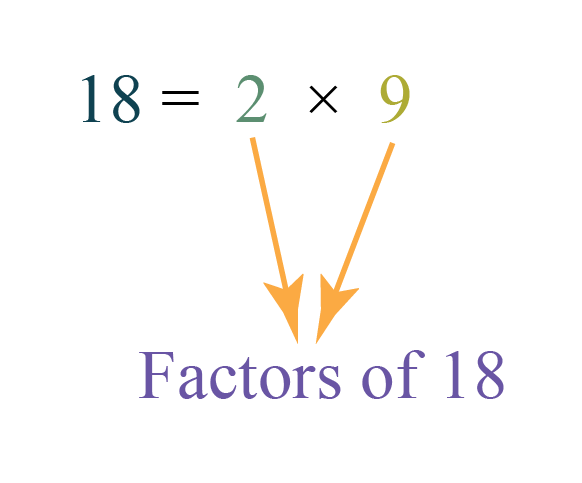Similarly, $$18$$ can be written as the products of the following numbers:

\begin{align} 18 &= 1 \times 18 \\ 18 &= 3 \times 6 \end{align}

Thus, the factors of $$18$$ are $$1,2,3,6,9$$ and $$18$$.

### Common Factors

A common factor of two or more numbers is a factor that is common to both of the given numbers.

For example, the common factors of two numbers $$18$$ and $$27$$ can be found by listing the factors of both numbers.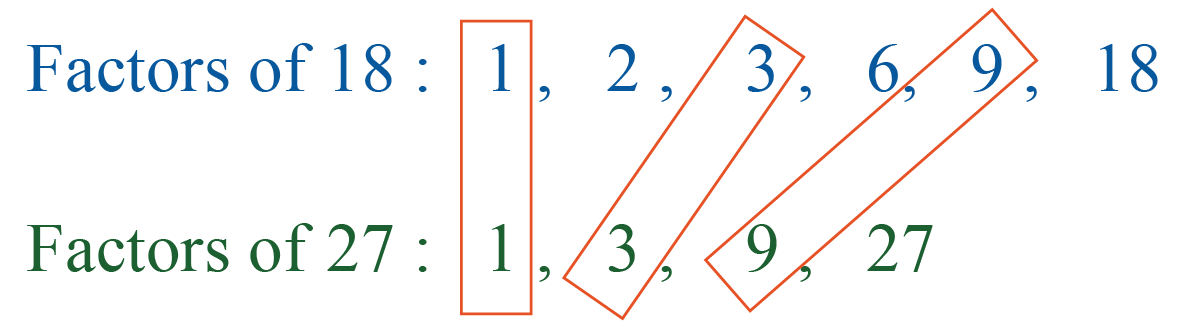Thus, the common factors of $$18$$ and $$27$$ are $$1,3$$ and $$9$$.

### HCF (Highest Common Factor)

• The HCF (Highest Common Factor) of two or more numbers is the highest number among all the common factors of the given numbers.

• The HCF (Highest Common Factor) of two natural numbers $$x$$ and $$y$$ is the largest possible number which divides both $$x$$ and $$y$$.

From the above example, the common factors of $$18$$ and $$27$$ are $$1,3$$ and $$9$$.

Among these numbers, $$9$$ is the highest (largest) number.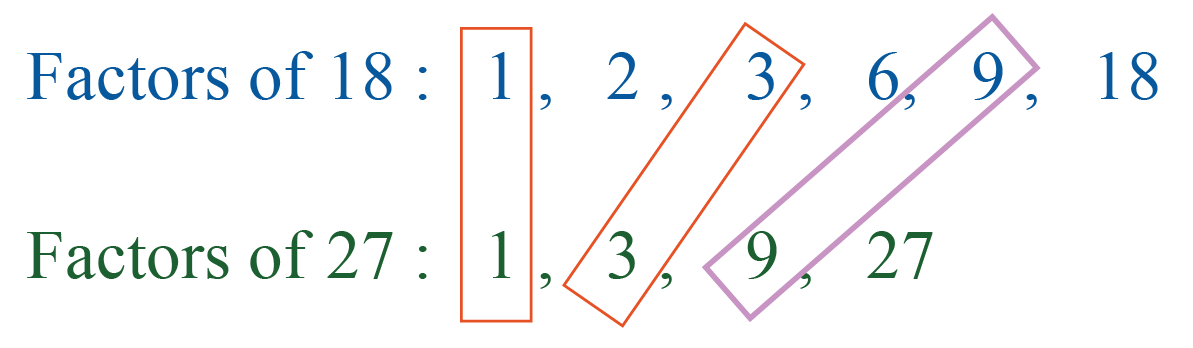Thus, the HCF of $$18$$ and $$27$$ is $$9$$.

This is written as:

$\text{HCF (18,27)} = 9$

## Learn These Along with HCF!

Here are a few more lessons related to the HCF.

These topics will not only help you master the concept for the highest common factor, but also other topics related to it.

 Check out  other related topics. Clcik on  any of the short lessons you want to explore Factors Multiples Composite Numbers Co-prime numbers Least Common Multiple Prime Factorization Prime Number

### HCF Examples

Using the above definition, the HCF of:

• $$60$$ and $$40$$ is $$20$$, i.e,
$\text { HCF} (60,40) = 20$
• $$100$$ and $$150$$ is $$50$$, i.e,
$\text { HCF} (150,50) = 50$
• $$144$$ and $$24$$ is $$24$$, i.e,
$\text { HCF} (144,24) = 24$
• $$17$$ and $$89$$ is $$1$$, i.e,
$\text { HCF} (17,89) = 1$

### Methods of Finding HCF

There are 3 methods to calculate the HCF of two numbers:

1. HCF by listing out the common factors
2. HCF by prime factorization
3. HCF by division method

Let us discuss each method in detail.

## 1. HCF by Listing out the Common Factors

Let us understand this method using the example below.

Example:

What is the HCF of $$30$$ and $$42$$?

Solution:

Step 1 - List the factors of each number.

Factors of $$30$$ - $$1, 2, 3, 5, 6, 10, 15, 30$$

Factors of $$42$$ - $$1, 2, 3, 6, 7, 14, 21, 42$$

Step 2 - Circle all the common factors.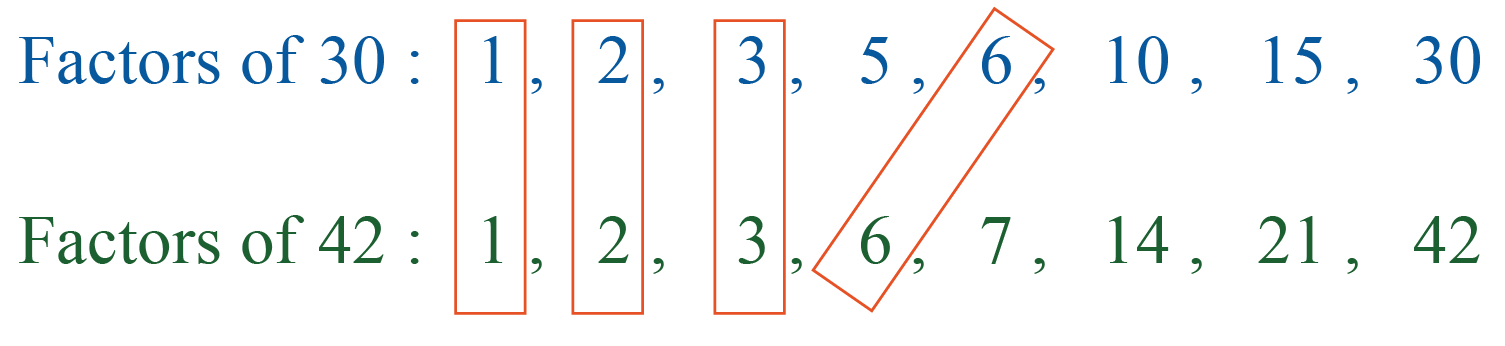Step 3 - $$6$$ is the common factor and the greatest one.

Hence, the HCF of $$30$$ and $$42$$ is $$6$$.

## 2. HCF by Prime Factorisation

Let us learn this method using the examples below.

Example 1:

What is the HCF of $$60$$ and $$90$$?

Solution:

Step 1 - Represent the numbers in the prime factored form.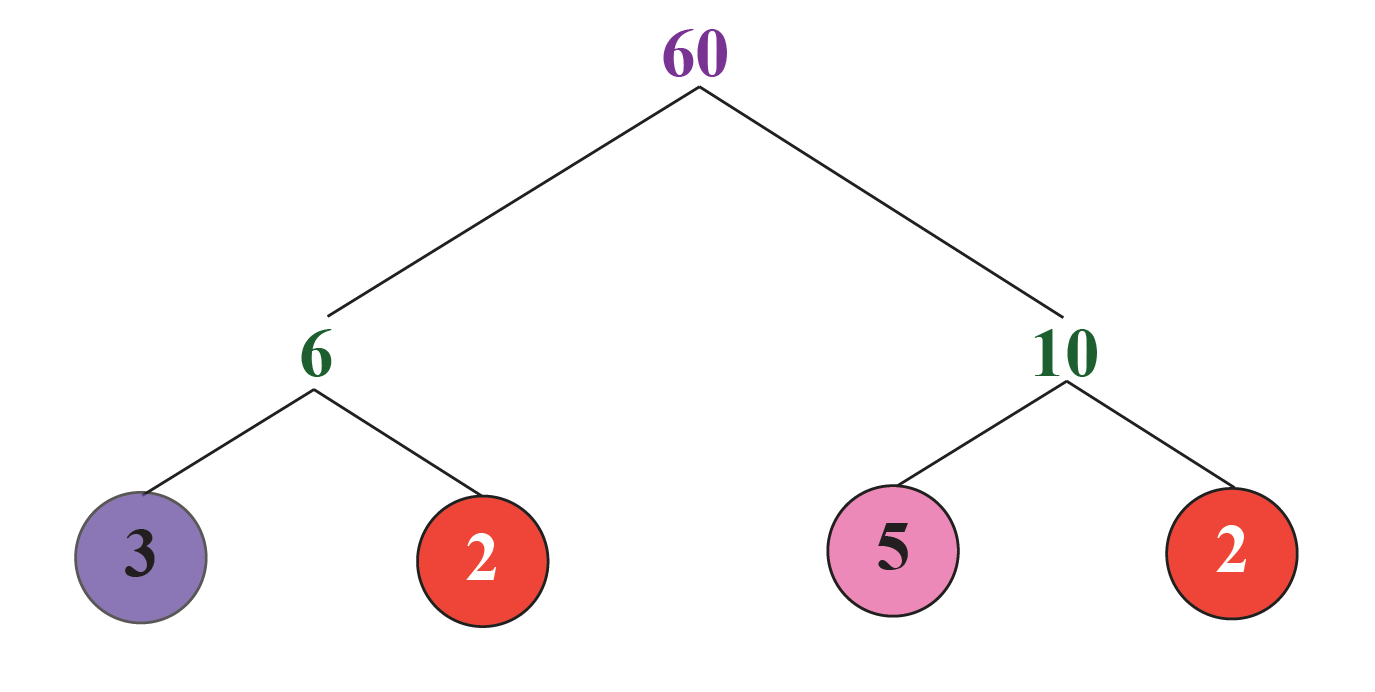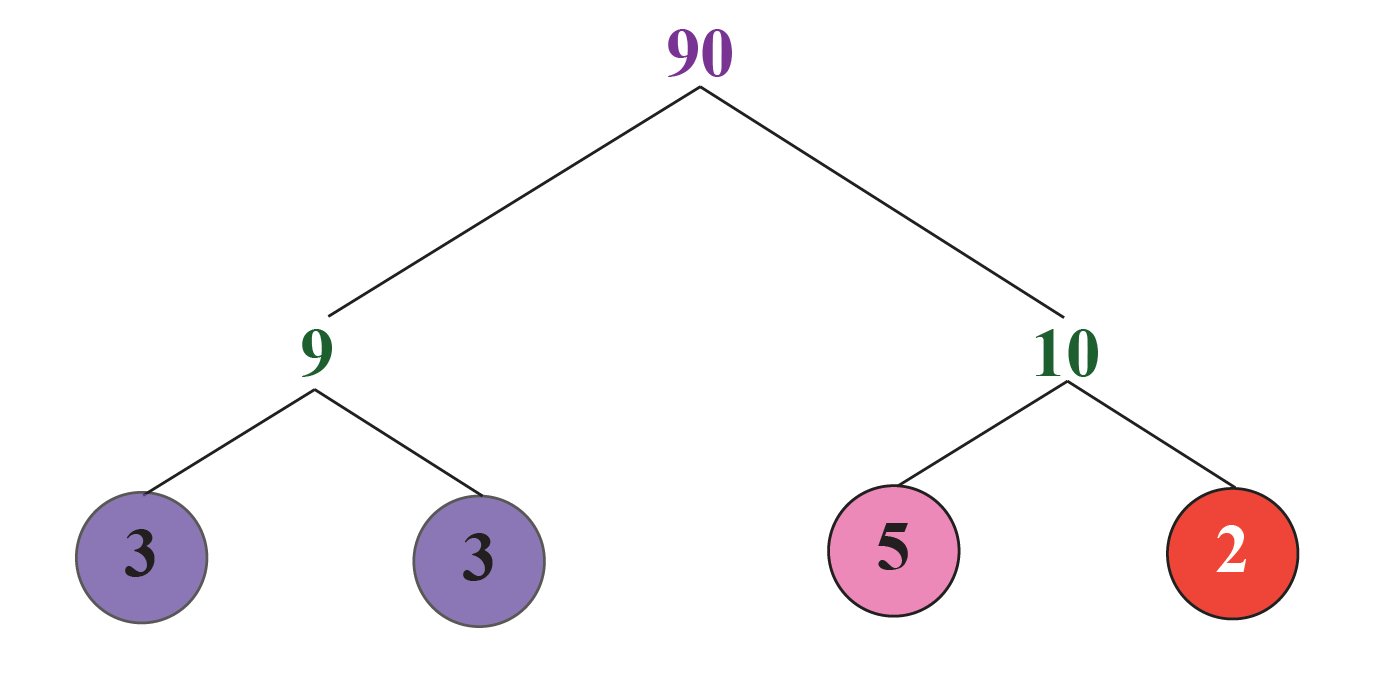Thus,

\begin{align} 60&= 2 \times 2 \times 3 \times 5\\ 90&=2 \times 3\times 3 \times 5 \end{align}

Step 2 - HCF is the product of the factors that are common to each of the given numbers.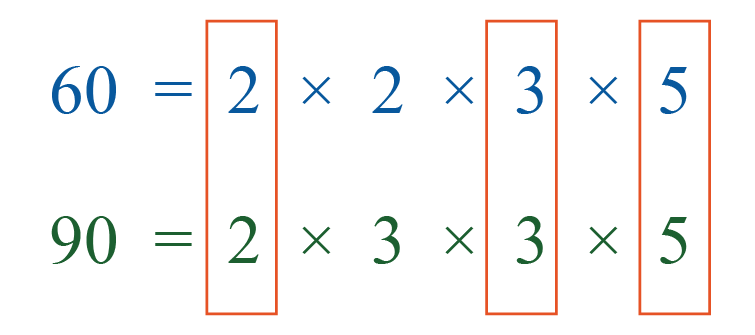This may seem confusing to you. Let us look at the following Venn diagram to understand this method better.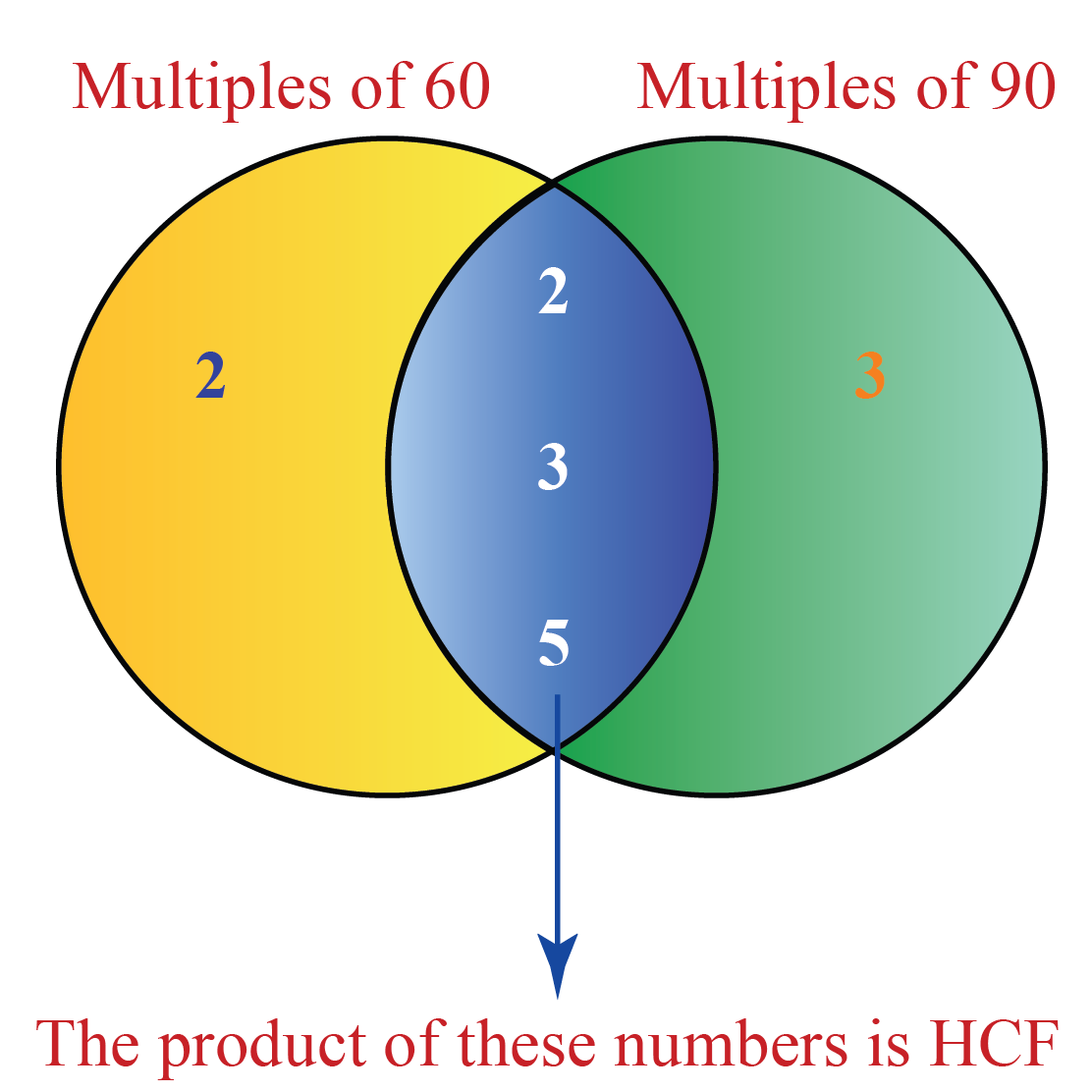Thus, HCF of $$60$$ and $$90$$ = $$2 \times 3 \times 5$$ = $$30$$.

Hence,

 HCF of 60 and 90 = 30

Example 2:

What is the HCF of $$120$$ and $$144$$?

Solution:

Step 1 - Represent the numbers in the prime factored form.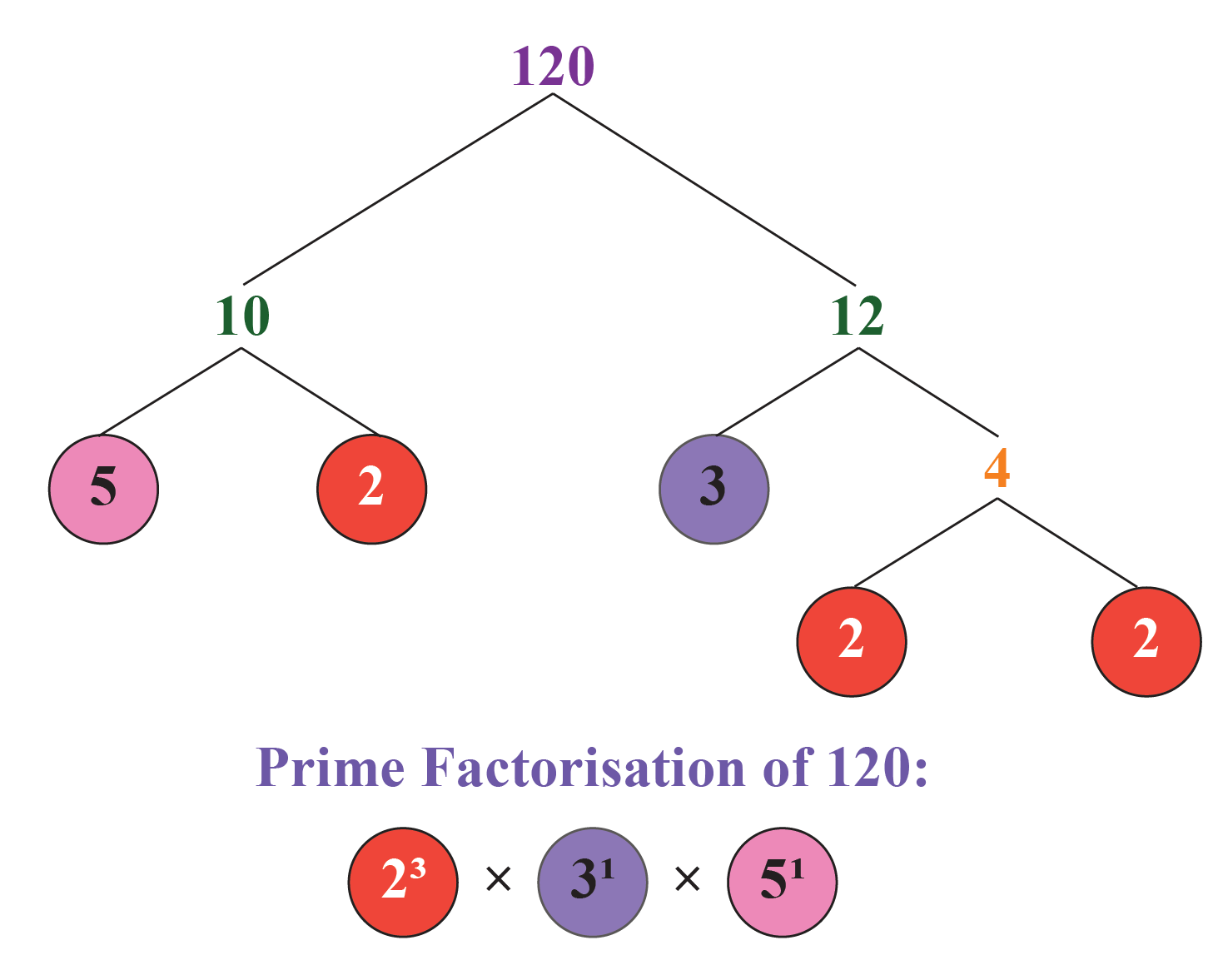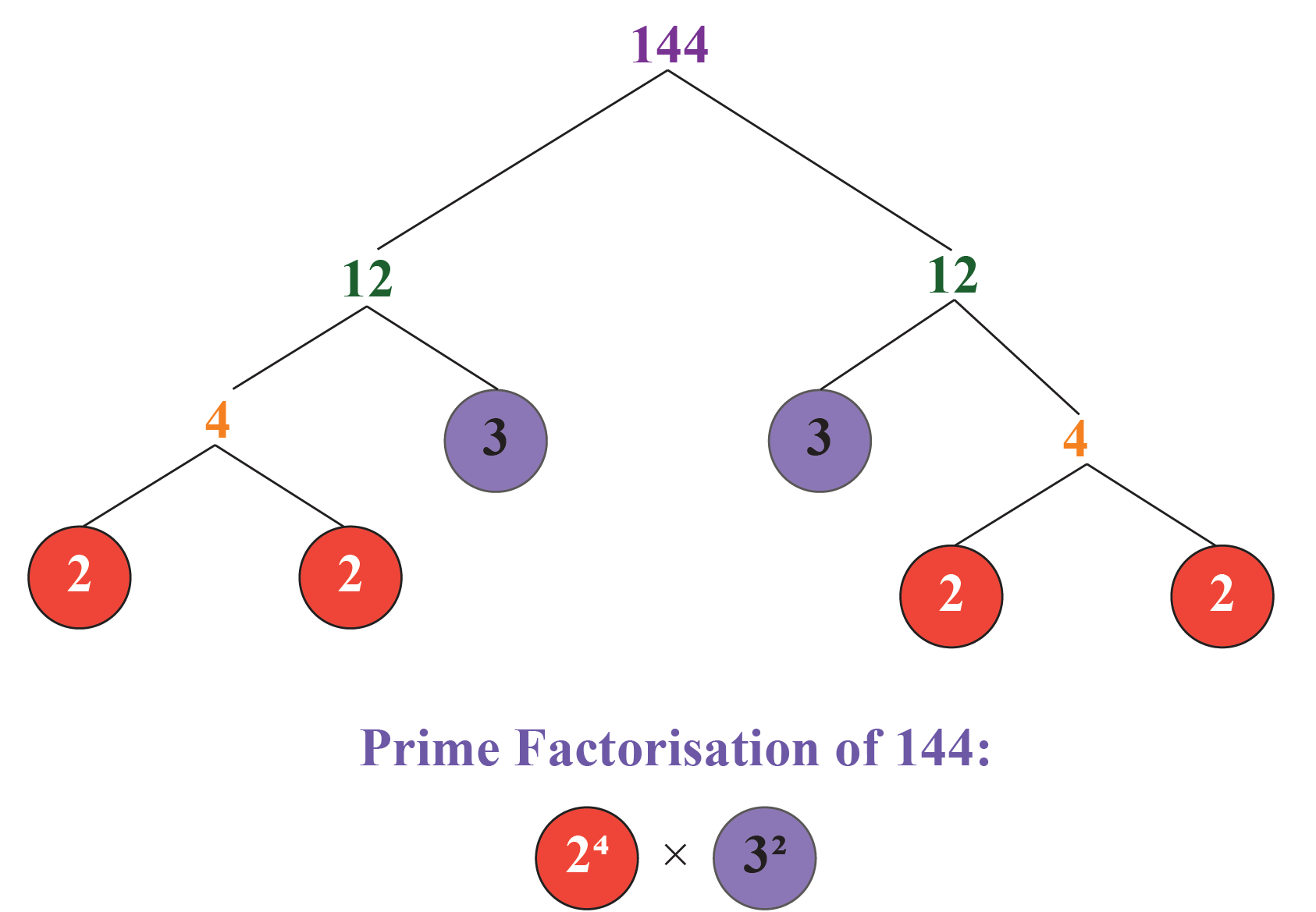Thus,

\begin{align} 120&=2^{3} \times 3^{1} \times 5^{1}\\ 144&=2^{4} \times 3^{2} \end{align}

Step 2 - HCF is the product of the smallest power of each common prime factor:

Thus,

$\mathrm{HCF}(120, 144)=2^{3} \times 3^{1} = 24$

Hence,

 HCF of 120 and 144 = 24

## 3. HCF by Division Method

Let us learn this method using the example below.

Example:

Find the HCF of $$198$$ and $$360$$ using the division method.

Solution:

Among the given two numbers, $$360$$ is the larger number, and $$198$$ is the smaller number.

Step 1 - Divide the larger number by the smaller number using long division.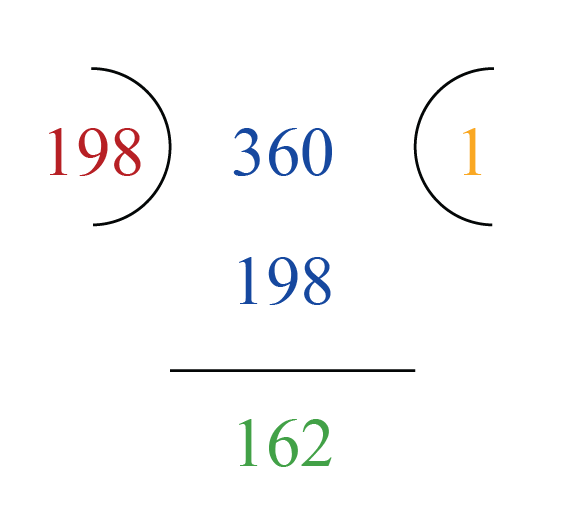Step 2 - If the remainder is $$0$$, then the divisor is the HCF.

If the remainder is NOT $$0$$, then make the remainder of the above step as the divisor and the divisor of the above step as the dividend and perform long division again.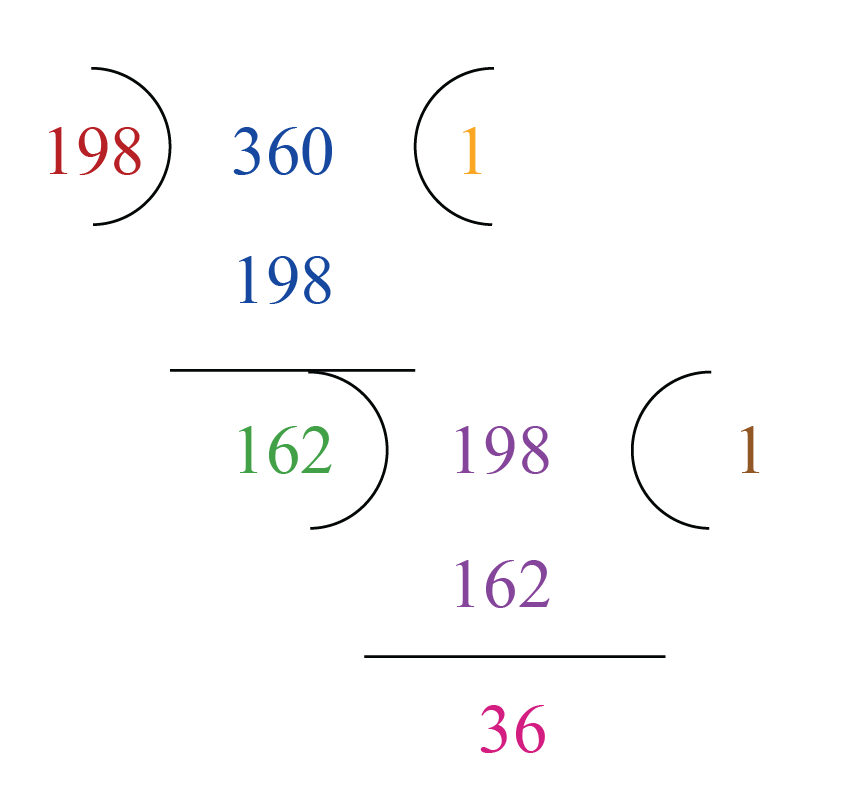Step 3 - If the remainder is $$0$$, then the divisor of the last division is the HCF.

If the remainder is NOT $$0$$, then we have to repeat step 2 until we get the remainder as $$0$$.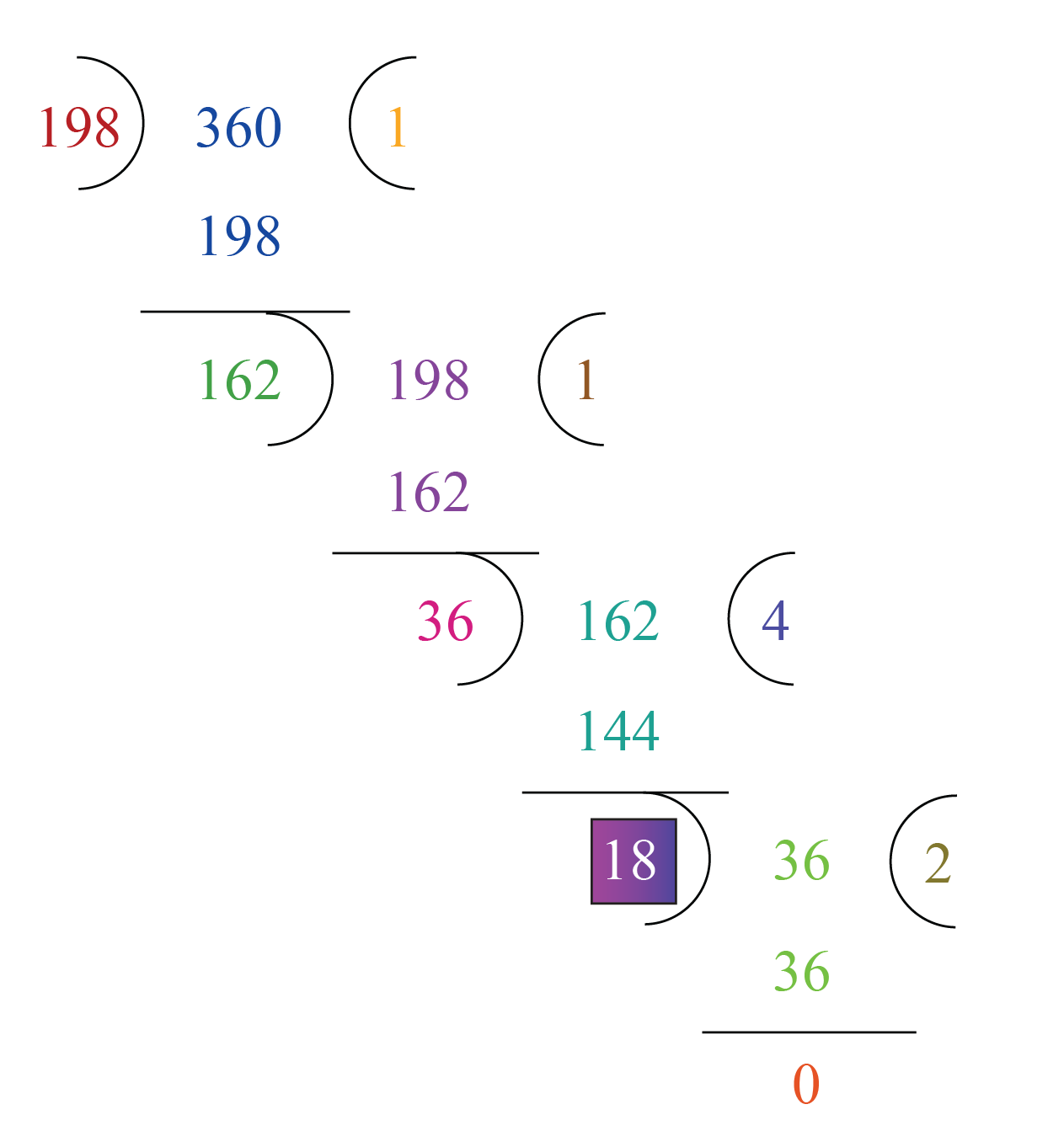Therefore, the HCF of the given two numbers is the divisor of the last division.

In this case, the divisor of the last division is $$18$$.

Thus,

 HCF of 198 and 360 = 18

### HCF of Multiple Numbers

The process of finding the HCF of multiple numbers is the same as explained above for the methods “HCF by listing out the common factors” and “HCF by prime factorisation.”

However, the method to find the HCF of multiple numbers by division method is different.

1. To find the HCF of three numbers:

• First, we will find the HCF of two of the numbers.
• Next, we will find the HCF of the third number and the HCF of the first two numbers.

Example:

Find the HCF of $$126, 162$$ and $$180$$.

Solution:

First, we will find the HCF of the two numbers $$126$$ and $$162$$.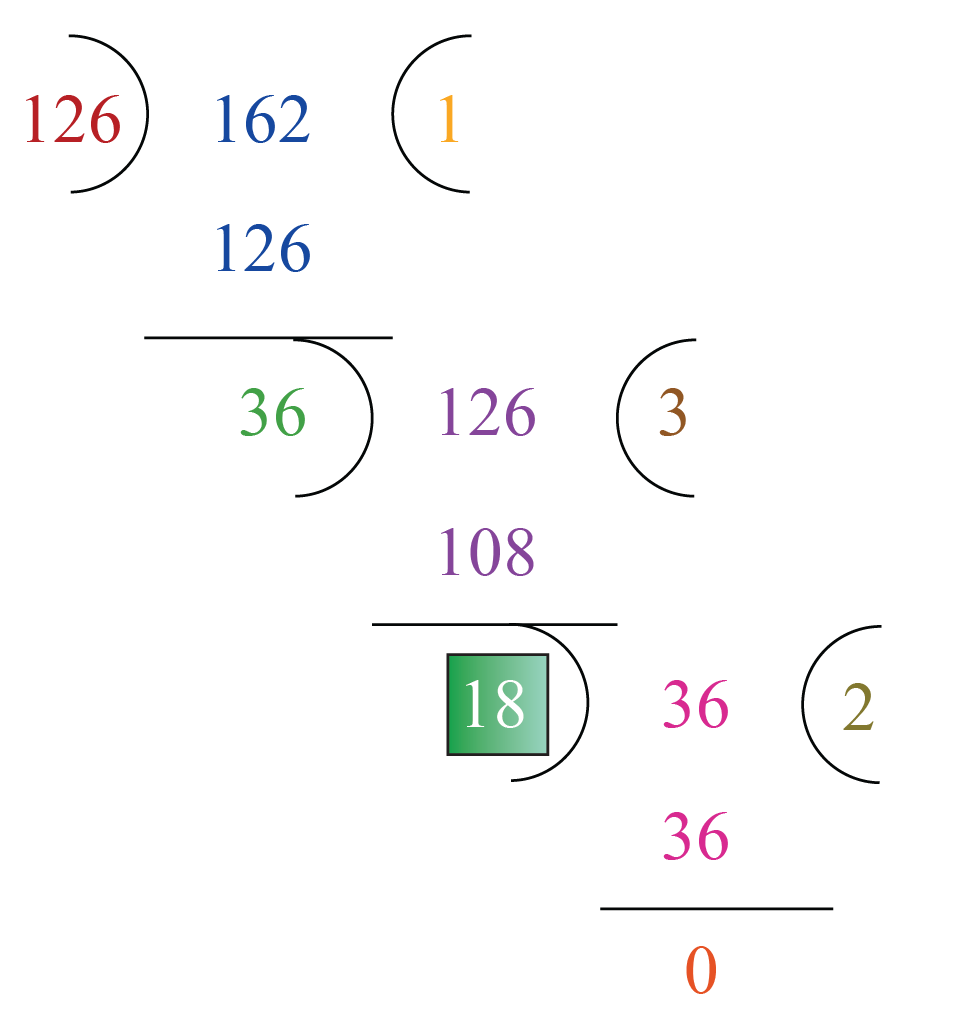Thus,

$\text{HCF of 126 and 162}=18 \,\,\,\,\, \rightarrow (1)$

Next, we will find the HCF of the third number, which is $$180$$, and the above HCF $$18$$.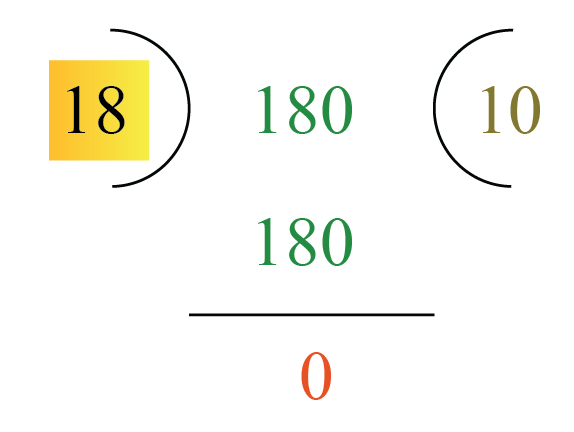Thus,

$\text{HCF of 180 and 18}=18 \,\,\,\,\, \rightarrow (2)$

From (1) and (2),

 HCF of $$126, 162$$ and $$180$$ is $$18$$

2. To find the HCF of four numbers:

• First, we will find the HCF of the two pairs of numbers separately.
• Next, we will find the HCF of the HCFs of the two pairs of numbers.

Example:

Find the HCF of $$72,140,256$$ and $$24$$.

Solution:

First, we will find the HCF of the first two numbers $$72$$ and $$140$$.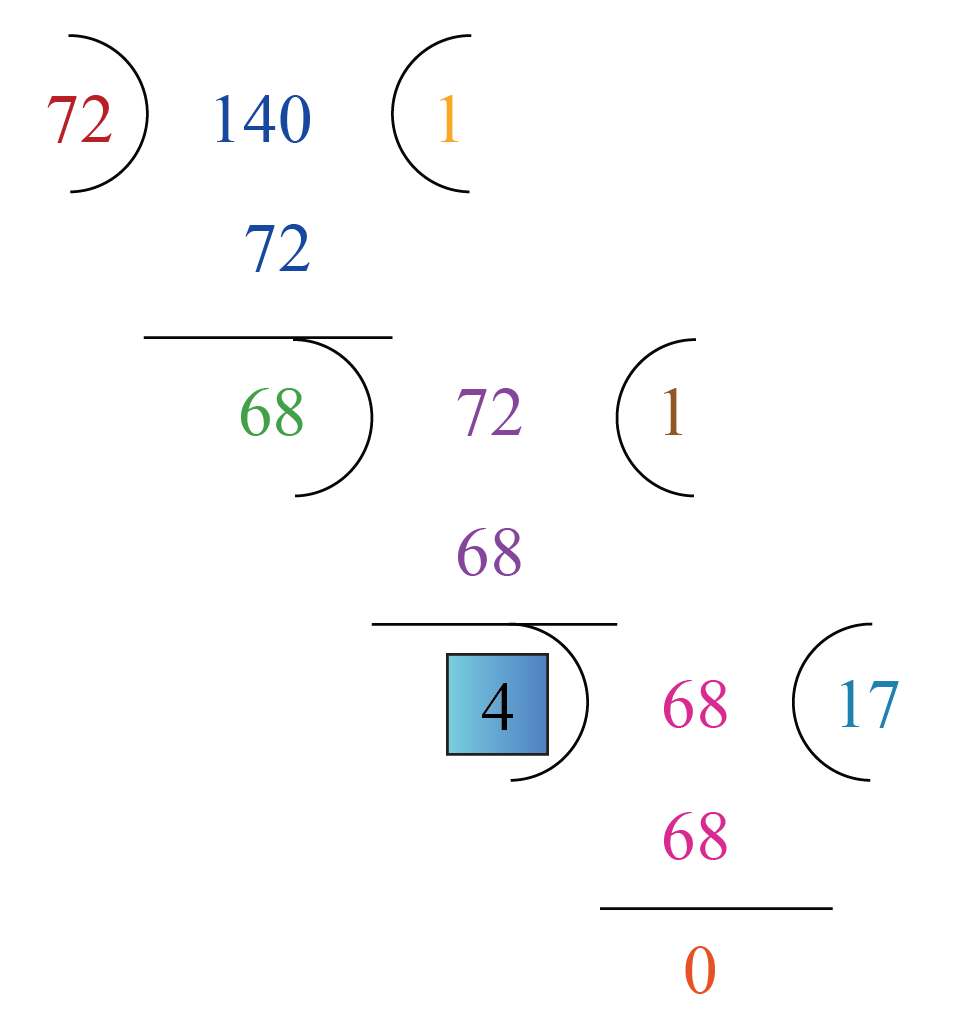Thus,

$\text{HCF of 72 and 140}=4 \,\,\,\,\, \rightarrow (1)$

Next, we will find the HCF of the last two numbers $$256$$ and $$24$$.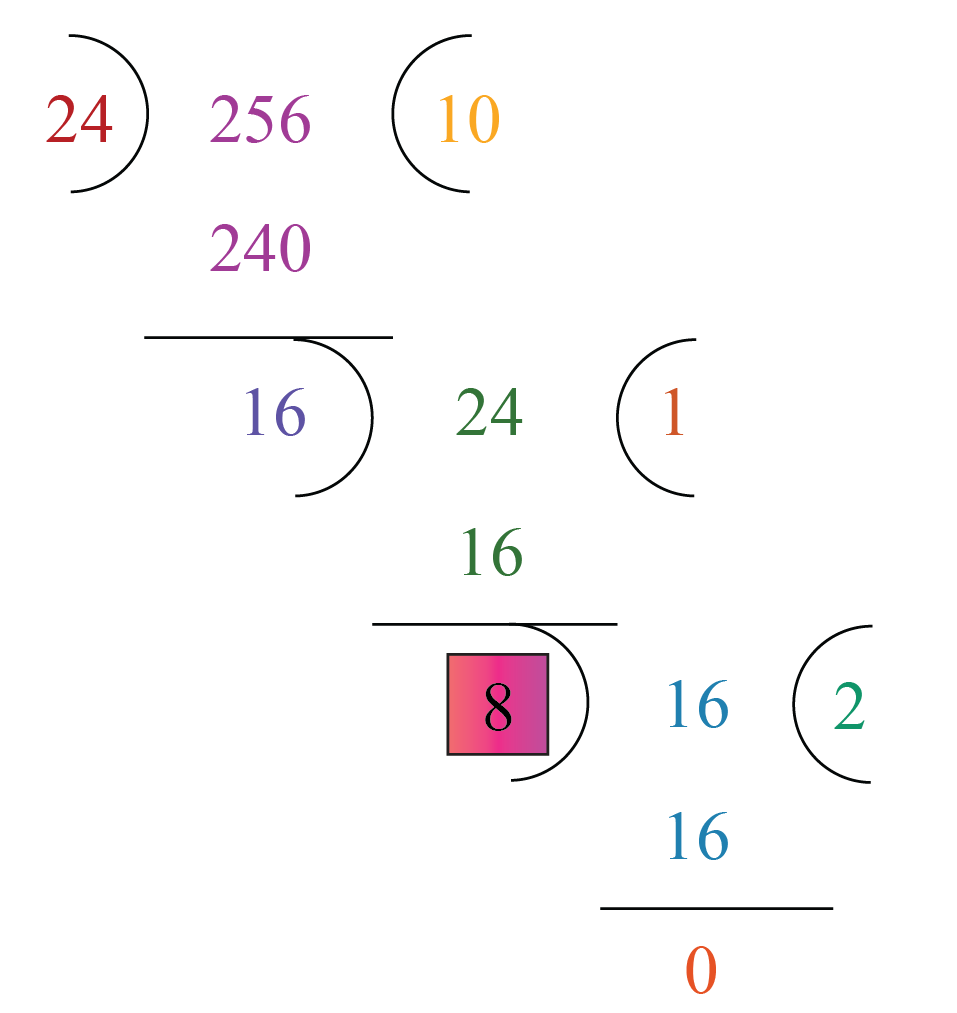Thus,

$\text{HCF of 256 and 24}=8 \,\,\,\,\, \rightarrow (2)$

Now, we will find the HCF of the HCFs that are in equation (1) and equation (2), i.e., we will find the HCF of $$4$$ and $$8$$.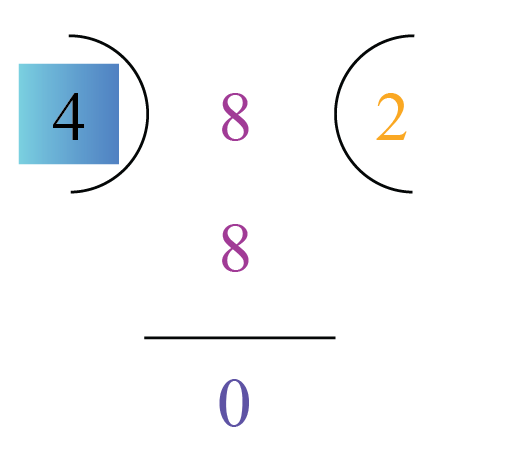Thus,

$\text{HCF of 4 and 8}=4$

 HCF of $$72,140,256$$ and $$24$$ is $$4$$

### HCF of Prime Numbers

We know that a prime number has only two factors, $$1$$ and itself.

Let us consider two prime numbers $$2$$ and $$7$$, and find their HCF by listing their factors.

Factors of $$2$$ are $$1, 2$$.

Factors of $$7$$ are $$1, 7$$.

We can see that the only common factor of $$2$$ and $$7$$ is $$1$$ and hence, this is the HCF.

Thus,

 HCF of prime numbers = $$1$$Challenging Questions
1. Jibin purchases two bags of cement weighing 105 Kg and 120 kg. Find the maximum value of weight which can measure the weight of the cement exact number of times.
2. Three tankers contain 168 liters, 336 liters, and 448 liters of petrol respectively. Find the maximum capacity of a container that can measure the petrol of the three containers an exact number of times.

### Highest Common Factor (HCF) Calculator

The following "Highest Common Factor Calculator" would show the HCF of the numbers that you enter.

Enter the numbers you wish to find the HCF of and click on the Go button:

## Solved Examples on HCF

 Example 1

Find the HCF of $$6, 72$$ and $$120$$ by using the "listing factors" method.

Solution:

The given numbers are $$6, 72$$ and $$120$$.

We will find the factors of each of these numbers.

Factors of $$6$$ = $$1, 2, 3, 6$$

Factors of $$72$$ = $$1, 2, 3, 4, 6, 8, 9, 12, 18, 24, 36, 72$$

Factors of $$120$$ = $$1, 2, 3, 4, 5, 6, 8, 10, 12, 15, 20, 24, 30, 40, 60, 120$$

Now, circle the common factors.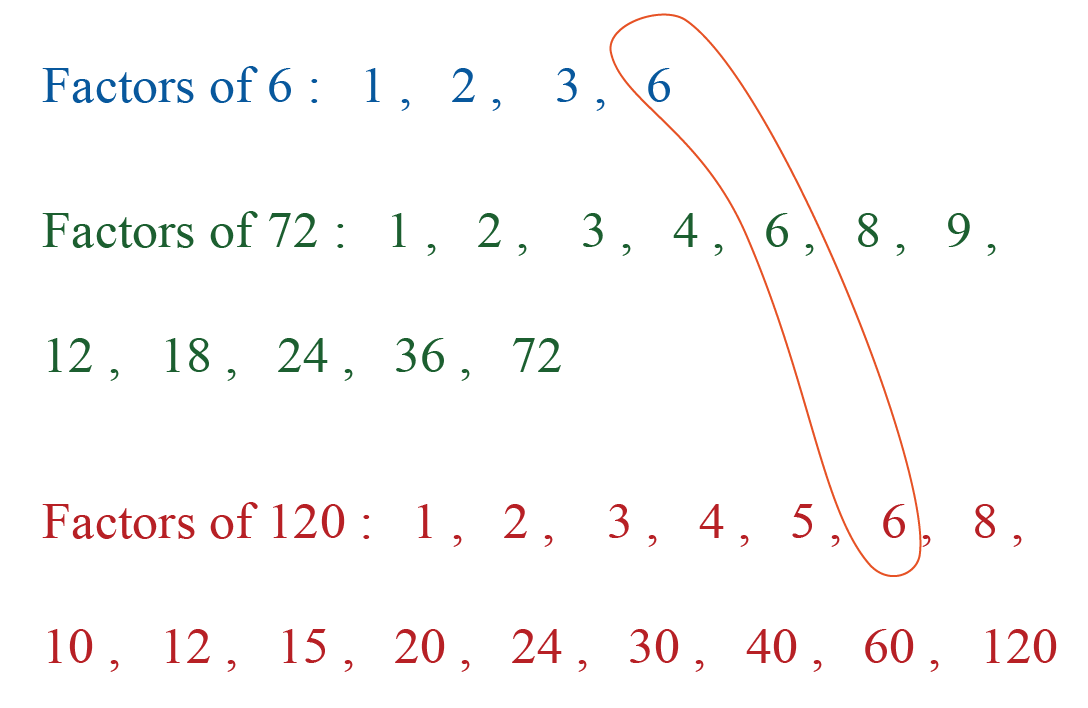$$6$$ is the only common factor and hence, it is the HCF.

 HCF of $$6, 72$$ and $$120$$ = $$6$$
 Example 2

Find the HCF of $$168, 252$$ and $$288$$ by the "prime factorisation method."

Solution:

The given numbers are $$168, 252$$ and $$288$$.

We will find the prime factorisation of each of these numbers.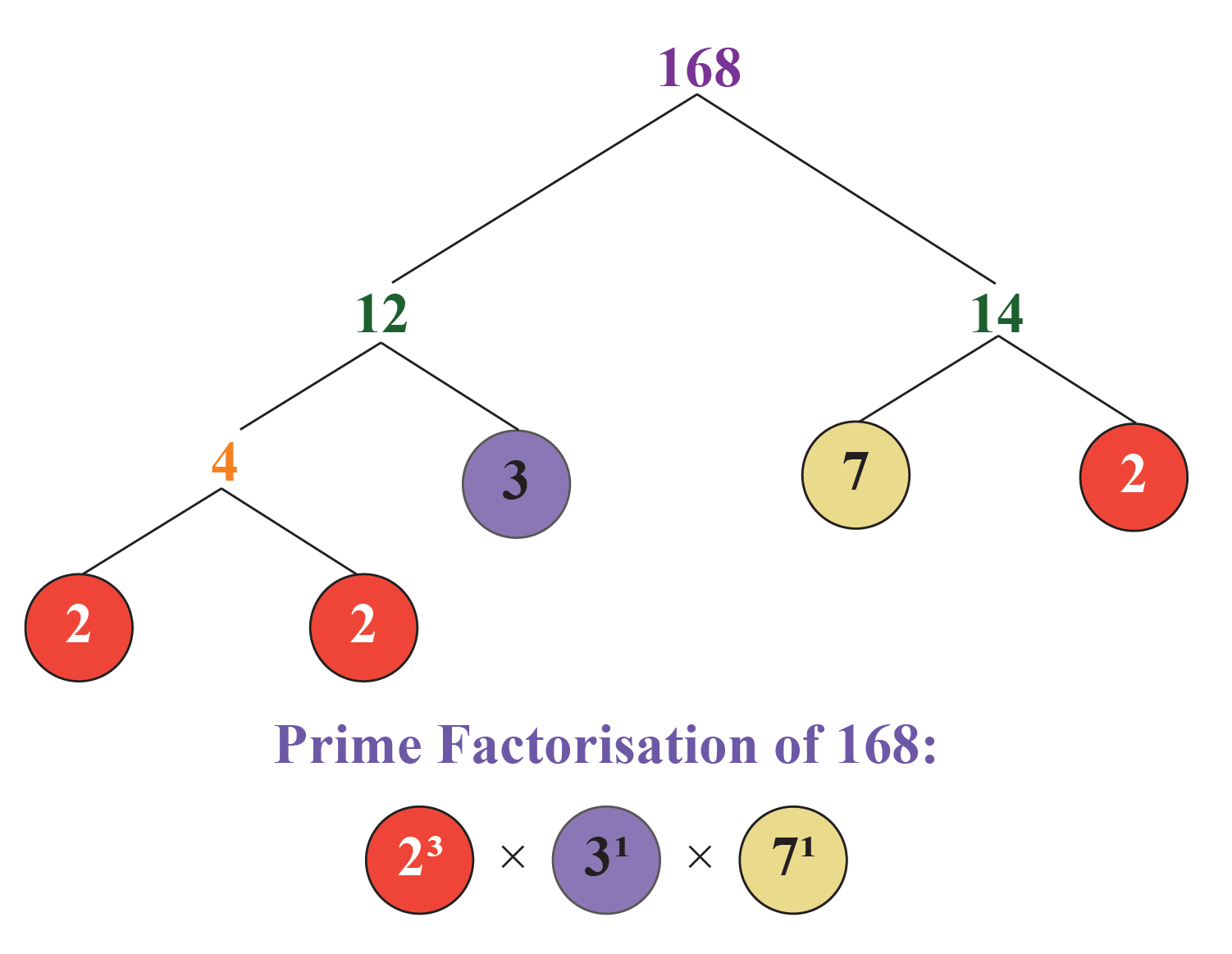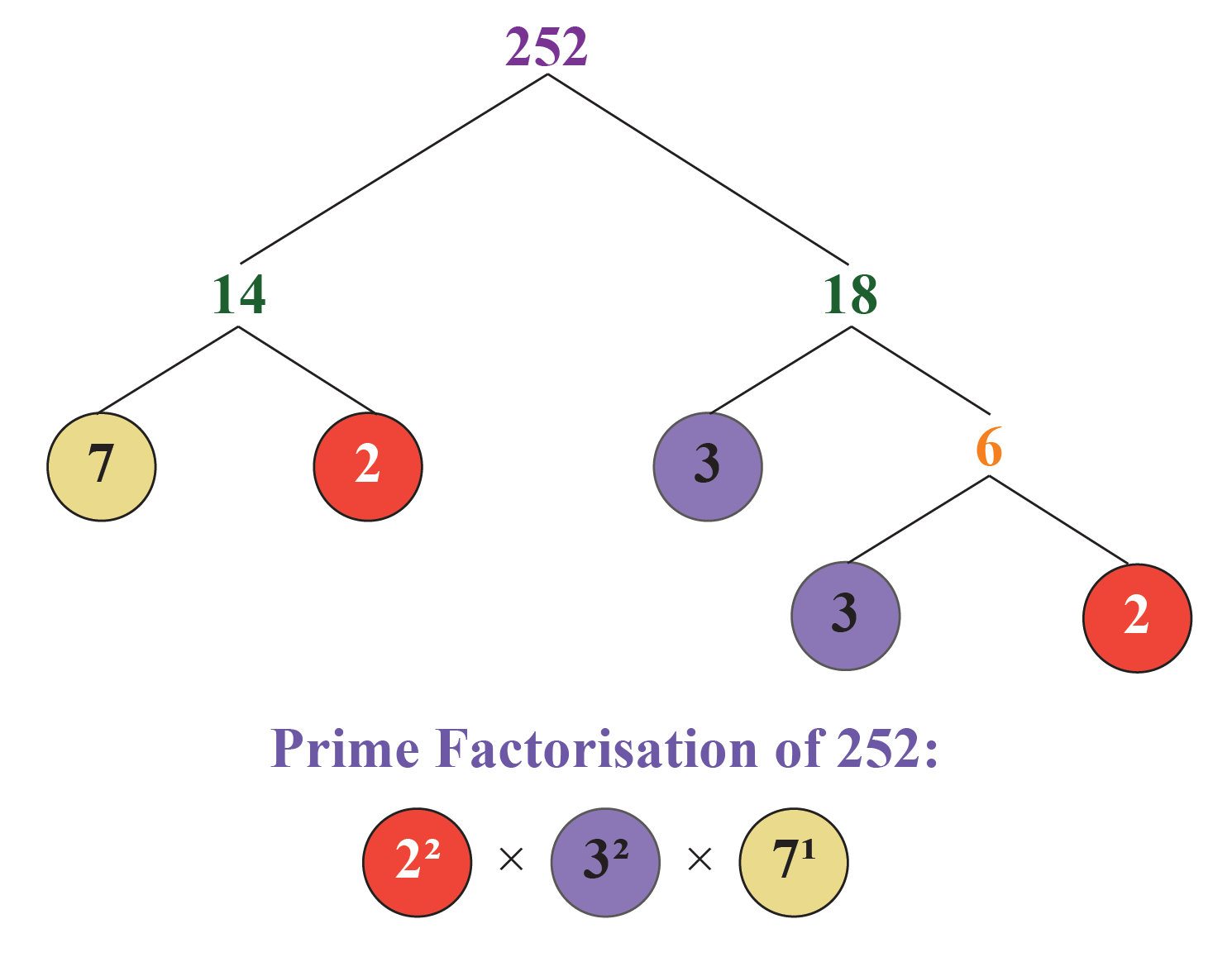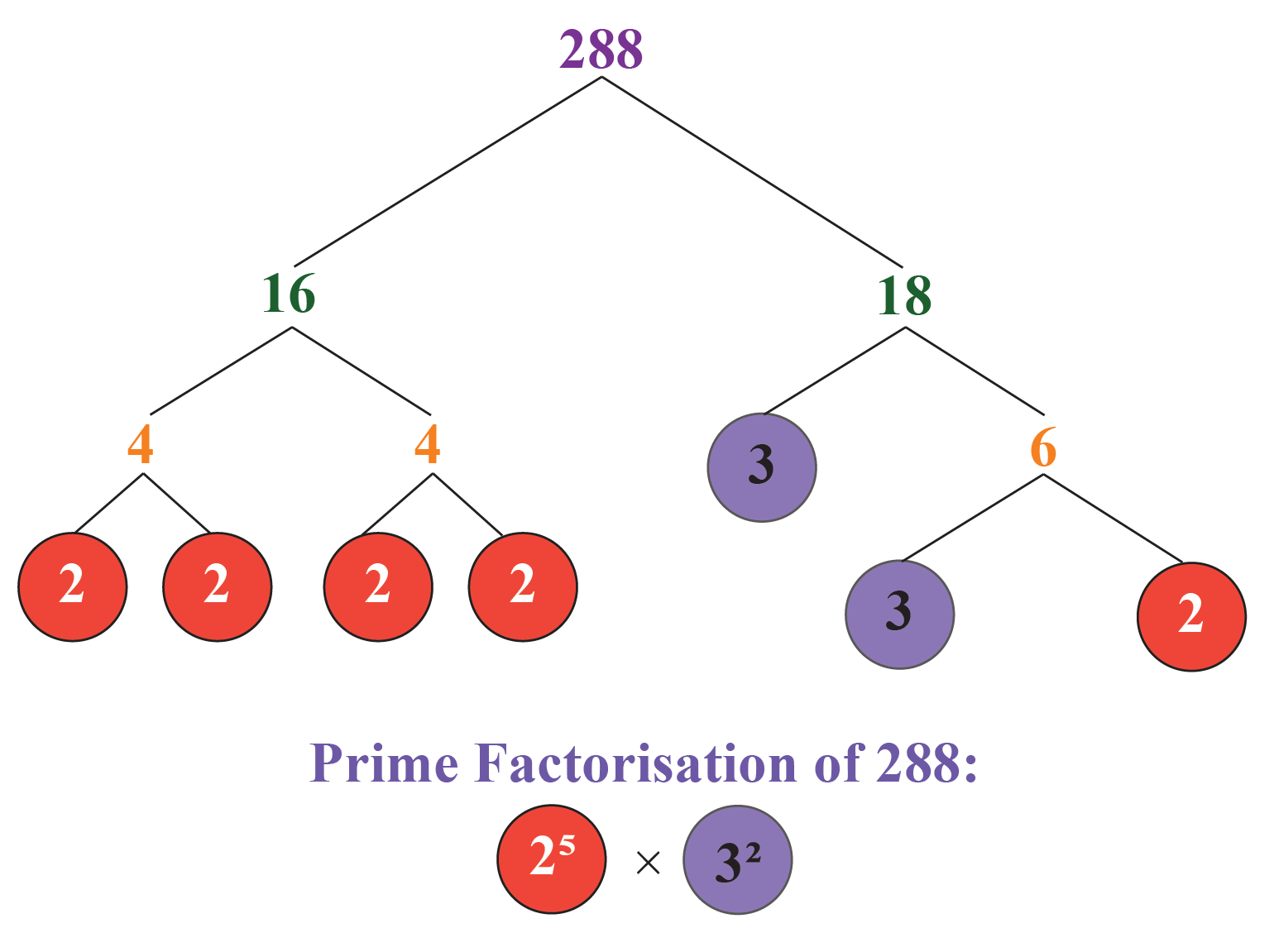Thus,

\begin{align}168&=2^{3} \times 3^{1} \times 7^{1}\\[0.3cm]252&=2^{2} \times 3^{2} \times 7^{1}\\[0.3cm]288&=2^{5} \times 3^{2}\end{align}

The HCF of these three numbers will be the product of the smallest power of each common prime factor of the three numbers.

Thus,

$\text{HCF of 168, 252 and 288} = 2^2 \times 3^1 = 12$

Hence,

 HCF of $$168, 252$$ and $$288$$ = 12
 Example 3

Find the HCF of $$9000$$ and $$980$$ using the "division method."

Solution:

Among the given numbers, $$9000$$ is the smallest, and $$980$$ is the largest.

We will divide the larger number by the smaller number.

Next, we will make the remainder as the divisor and the last divisor as the dividend and divide again.

We will repeat this process until the remainder is $$0$$.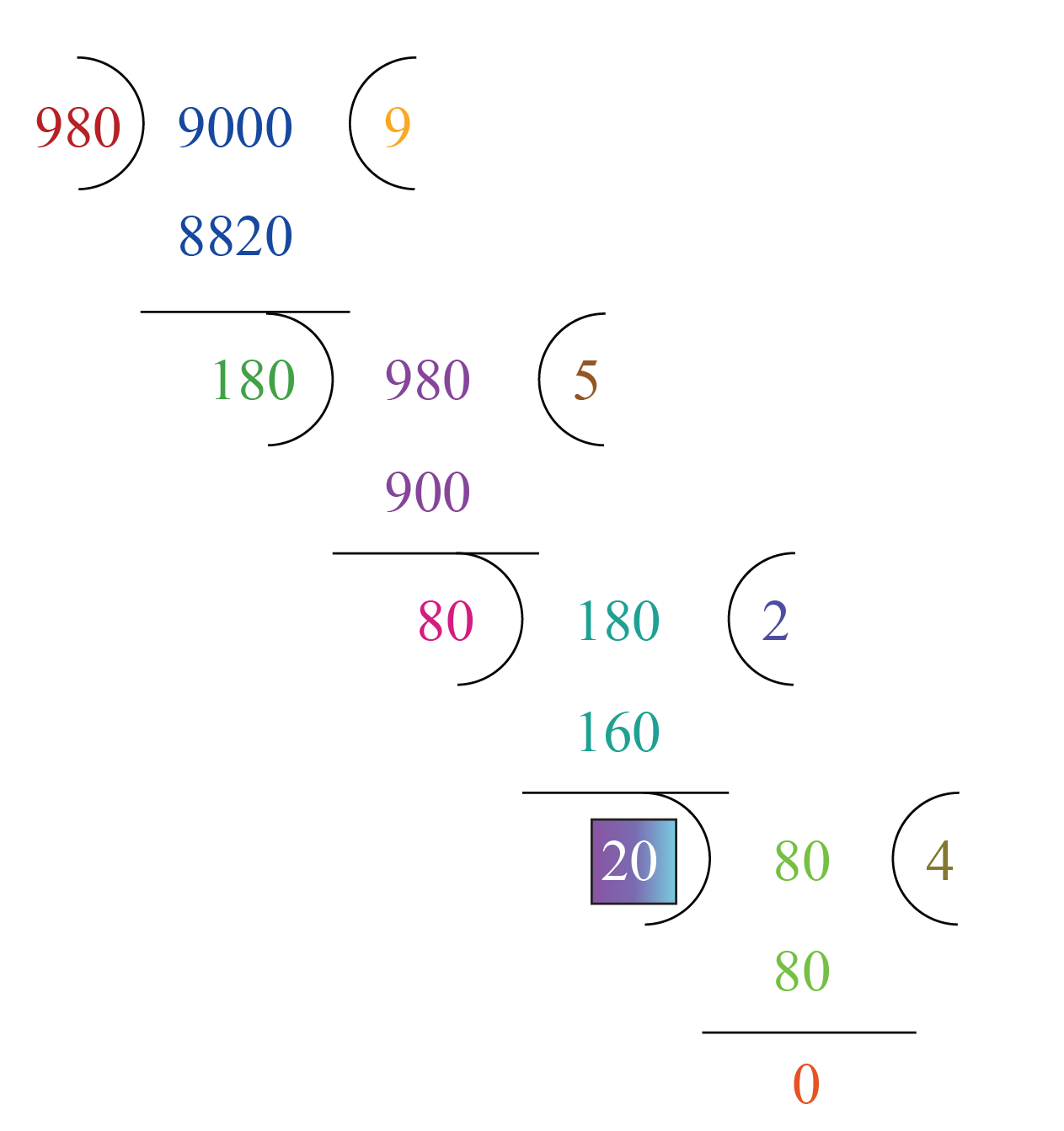Thus,

 HCF of $$9000$$ and $$980$$ = $$20$$Think Tank
1. Two positive numbers $$a$$ and $$b$$ are written as $$a=x^3y^2$$  & $$b=xy^3$$; $$x$$ and $$y$$ are prime numbers. Find HCF $$(a,b)$$.
2. What is the HCF of coprime numbers?

## Interactive Questions on HCF

Here are a few activities for you to practice. Select/Type your answer and click the "Check Answer" button to see the result.

## Summary

The magic of math lies in the amazing concepts that it is built upon. At Cuemath, we explore and interact with these concepts in a fun way!

The math journey around HCF starts with the basics of numbers and goes on to creatively crafting a fresh concept in the young minds. Done with real-life and relatable examples.

The best part, this isn’t the end. With a universe built around HCF at Cuemath, one can take their math journey forward with solved examples, practice questions, quizzes, worksheets, practice papers, and so much more. Again, all of it, exclusively around the Highest Common Factor.

## FAQs on HCF

### What is the Highest Common Factor?

• The HCF (Highest Common Factor) of two or more numbers is the highest number among all the common factors of the given numbers.

• The HCF (Highest Common Factor) of two natural numbers $$x$$ and $$y$$ is the largest possible number which divides both $$x$$ and $$y$$.

You can learn the definition of HCF and view the HCF examples under the "HCF (Highest Common Factor) Definition" section of this page.

### How do you Find the HCF in Math?

There are 3 methods to calculate the HCF of two numbers:

1. HCF by listing out the common factors
2. HCF by prime factorization
3. HCF by division method

These methods are explained in detail with examples under the section titled "Methods of Finding HCF" on this page.

### What are the Properties of HCF?

The properties of HCF are:

• HCF of two or more numbers divides each of the numbers without a remainder.
• HCF of two or more numbers is a factor of each of the numbers.
• HCF of two or more numbers is always less than or equal to each of the numbers.
• HCF of two or more prime numbers is $$1$$ always.

### Where Can I Find the HCF Calculator?

We can find the HCF calculator under the "Highest Common Factor Calculator" section of this page.

You can enter a list of numbers in this calculator and it will show the HCF of the entered numbers.

## Free Worksheets on HCF

Arithmetic Integers
grade 10 | Questions Set 2
Arithmetic Integers
Arithmetic Integers
grade 10 | Questions Set 1
Arithmetic Integers
HCF and LCM
HCF and LCM